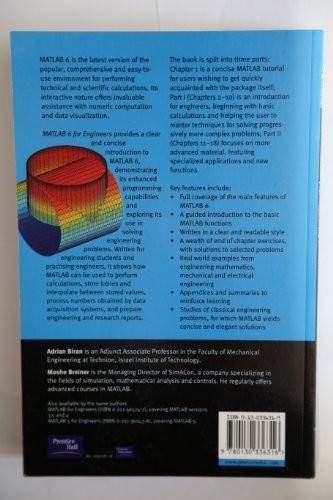# MATLAB 6 for Engineers by Adrian B. Biran

In Stock
\$20.99
+10% GST
Aims to simplify use of MATLAB 6 by walking the user through the main functions, facilities and applications. The text covers some of the new features, but does cover any of the advanced features in-depth. It is specifically geared towards the needs of engineering students who are expected to use MATLAB to model and solve real engineering problems.
Only 1 left

## MATLAB 6 for Engineers Summary

### MATLAB 6 for Engineers by Adrian B. Biran

As the features of MATLAB are becoming more advanced, the literature more confusing and the package harder to navigate, this new text will aim to simplify use of MATLAB 6 by walking the user through the main functions, facilities and applications. It will cover some of the new features, but won't cover any of the advanced features in-depth. Like the previous edition, it will be specifically geared towards the needs of engineering students who are expected to use MATLAB to model and solve real engineering problems.

### Why buy from World of BooksOur excellent value books literally don't cost the earthFree delivery in AustraliaEvery used book bought is one saved from landfill

1. MATLAB in a Nutshell.
2. Desktop Calculations with Graphics.
3. Two-Dimensional Arrays and Matrices.
4. Complex Numbers.
5. Geometric Calculations.
6. Solving Equations.
7. Programming in MATLAB.
8. External Files.
9. Regression and Interpolation.
11. Numerical Integration.
12. Graph Theory.
13. Dimensional Analysis.
14. System Modelling and Integration.
15. Control.
16. Signal Processing.
17. Case Studies in Mechanical Engineering.

GOR002694139
MATLAB 6 for Engineers by Adrian B. Biran